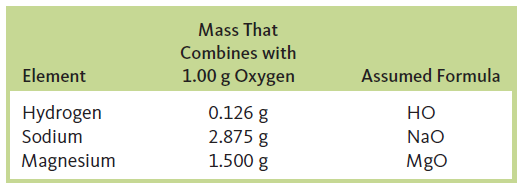# Problem: Early tables of atomic weights (masses) were generated by measuring the mass of a substance that reacts with 1.00 g of oxygen. Given the following data and taking the atomic mass of hydrogen as 1.00, generate a table of relative atomic masses for oxygen, sodium, and magnesium.

🤓 Based on our data, we think this question is relevant for Professor Fakhreddine's class at TEXAS.

###### FREE Expert Solution

We can use the mass ratio given in the table to calculate the mass of O when the mass of H = 1.00g. Since 1.00g stands for the atomic mass of Hydrogen, the calculated value will be the atomic mass of O as well. Take note that this should not match the values in the periodic table because this is the old system that they used to get a relative atomic mass and as we can see the assumed formula was wrong.###### Problem Details

Early tables of atomic weights (masses) were generated by measuring the mass of a substance that reacts with 1.00 g of oxygen. Given the following data and taking the atomic mass of hydrogen as 1.00, generate a table of relative atomic masses for oxygen, sodium, and magnesium.